Next: Sensitivity to initial conditions Up: The chaotic pendulum Previous: Period-doubling bifurcations

## The route to chaos

Let us return to Fig. 39, which tracks the evolution of a left-favouring periodic attractor as the quality-factor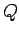is gradually increased. Recall that whenexceeds a critical value, which is about, then the attractor undergoes a period-doubling bifurcation which converts it from a period-1 to a period-2 attractor. This bifurcation is indicated by the forking of the curve in Fig. 39. Let us now investigate what happens as we continue to increase. Fig. 43 is basically a continuation of Fig. 39. It can be seen that, asis gradually increased, the attractor undergoes a period-doubling bifurcation at, as before, but then undergoes a second period-doubling bifurcation (indicated by the second forking of the curves) at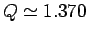, and a third bifurcation at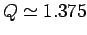. Obviously, the second bifurcation converts a period-2 attractor into a period-4 attractor (hence, two curves split apart to give four curves). Likewise, the third bifurcation converts a period-4 attractor into a period-8 attractor (hence, four curves split into eight curves). Shortly after the third bifurcation, the various curves in the figure seem to expand explosively and merge together to produce an area of almost solid black. As we shall see, this behaviour is indicative of the onset of chaos.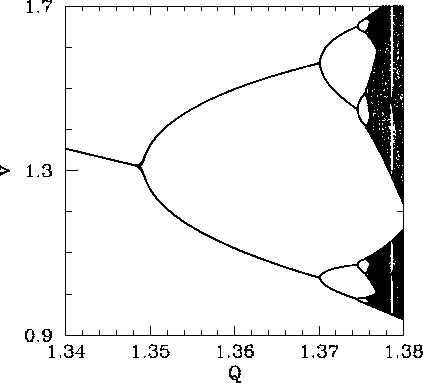Figure 44 is a blow-up of Fig. 43, showing more details of the onset of chaos. The period-4 to period-8 bifurcation can be seen quite clearly. However, we can also see a period-8 to period-16 bifurcation, at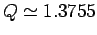. Finally, if we look carefully, we can see a hint of a period-16 to period-32 bifurcation, just before the start of the solid black region. Figures 43 and 44 seem to suggest that the onset of chaos is triggered by an infinite series of period-doubling bifurcations.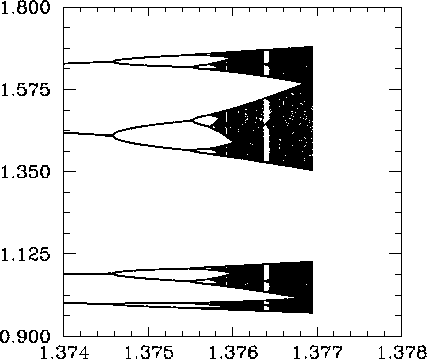Table 2 gives some details of the sequence of period-doubling bifurcations shown in Figs. 43 and 44. Let us introduce a bifurcation index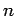: the period-1 to period-2 bifurcation corresponds to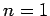; the period-2 to period-4 bifurcation corresponds to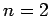; and so on. Let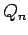be the critical value of the quality-factorabove which theth bifurcation is triggered. Table 2 shows the, determined from Figs. 43 and 44, forto 5. Also shown is the ratio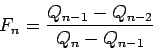(98)

for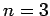to 5. It can be seen that Tab. 2 offers reasonably convincing evidence that this ratio takes the constant value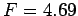. It follows that we can estimate the critical-value required to trigger theth bifurcation via the following formula: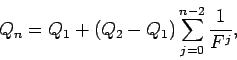(99)

for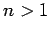. Note that the distance (in) between bifurcations decreases rapidly asincreases. In fact, the above formula predicts an accumulation of period-doubling bifurcations at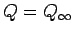, where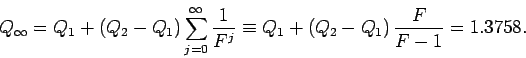(100)

Note that our calculated accumulation point corresponds almost exactly to the onset of the solid black region in Fig. 44. By the time thatexceeds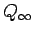, we expect the attractor to have been converted into a period-infinity attractor via an infinite series of period-doubling bifurcations. A period-infinity attractor is one whose corresponding motion never repeats itself, no matter how long we wait. In dynamics, such bounded aperiodic motion is generally referred to as chaos. Hence, a period-infinity attractor is sometimes called a chaotic attractor. Now, period-motion is represented byseparate curves in Fig. 44. It is, therefore, not surprising that chaos (i.e., period-infinity motion) is represented by an infinite number of curves which merge together to form a region of solid black.

 Bifurcation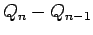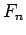period-1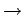period-2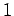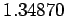- - period-2period-4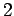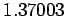0.02133 - period-4period-8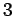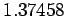0.00455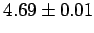period-8period-16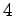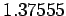0.00097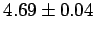period-16period-32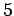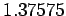0.00020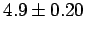Let us examine the onset of chaos in a little more detail. Figures 45-48 show details of the pendulum's time-asymptotic motion at various stages on the period-doubling cascade discussed above. Figure 45 shows period-4 motion: note that the Poincaré section consists of four points, and the associated sequence of net rotations per period of the pendulum repeats itself every four periods. Figure 46 shows period-8 motion: now the Poincaré section consists of eight points, and the rotation sequence repeats itself every eight periods. Figure 47 shows period-16 motion: as expected, the Poincaré section consists of sixteen points, and the rotation sequence repeats itself every sixteen periods. Finally, Fig. 48 shows chaotic motion. Note that the Poincaré section now consists of a set of four continuous line segments, which are, presumably, made up of an infinite number of points (corresponding to the infinite period of chaotic motion). Note, also, that the associated sequence of net rotations per period shows no obvious sign of ever repeating itself. In fact, this sequence looks rather like one of the previously shown periodic sequences with the addition of a small random component. The generation of apparently random motion from equations of motion, such as Eqs. (81) and (82), which contain no overtly random elements is one of the most surprising features of non-linear dynamics.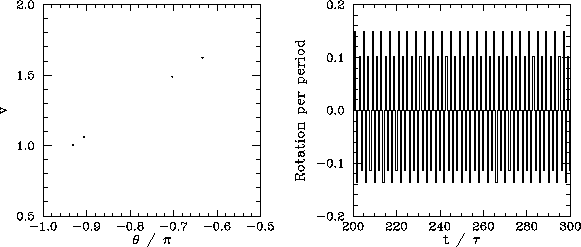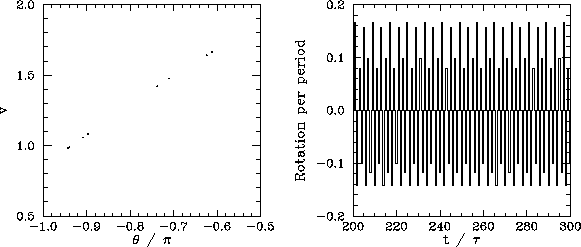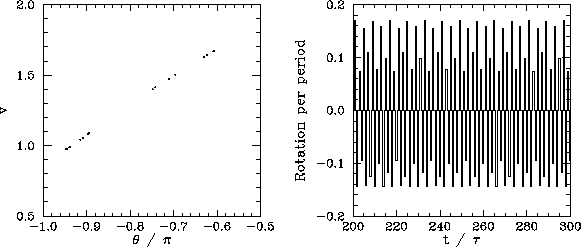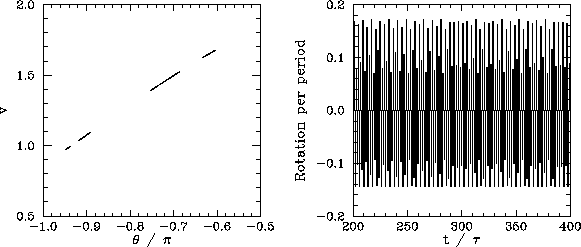Many non-linear dynamical systems, found in nature, exhibit a transition from periodic to chaotic motion as some control parameter is varied. Now, there are various known mechanisms by which chaotic motion can arise from periodic motion. However, a transition to chaos via an infinite series of period-doubling bifurcations, as illustrated above, is certainly amongst the most commonly occurring of these mechanisms. Around 1975, the physicist Mitchell Feigenbaum was investigating a simple mathematical model, known as the logistic map, which exhibits a transition to chaos, via a sequence of period-doubling bifurcations, as a control parameteris increased. Let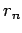be the value ofat which the first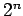-period cycle appears. Feigenbaum noticed that the ratio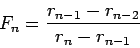(101)

converges rapidly to a constant value,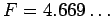, asincreases. Feigenbaum was able to demonstrate that this value of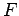is common to a wide range of different mathematic models which exhibit transitions to chaos via period-doubling bifurcations.28Feigenbaum went on to argue that the Feigenbaum ratio,, should converge to the value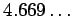in any dynamical system exhibiting a transition to chaos via period-doubling bifurcations.29 This amazing prediction has been verified experimentally in a number of quite different physical systems.30 Note that our best estimate of the Feigenbaum ratio (see Tab. 2) is, in good agreement with Feigenbaum's prediction.

The existence of a universal ratio characterizing the transition to chaos via period-doubling bifurcations is one of many pieces of evidence indicating that chaos is a universal phenomenon (i.e., the onset and nature of chaotic motion in different dynamical systems has many common features). This observation encourages us to believe that in studying the chaotic motion of a damped, periodically driven, pendulum we are learning lessons which can be applied to a wide range of non-linear dynamical systems.Next: Sensitivity to initial conditions Up: The chaotic pendulum Previous: Period-doubling bifurcations
Richard Fitzpatrick 2006-03-29